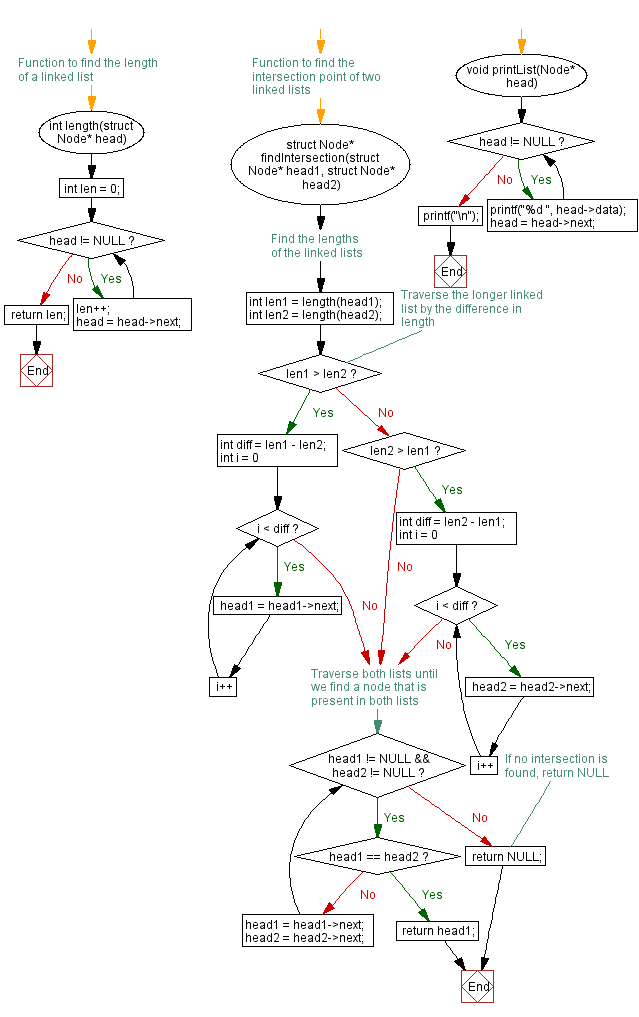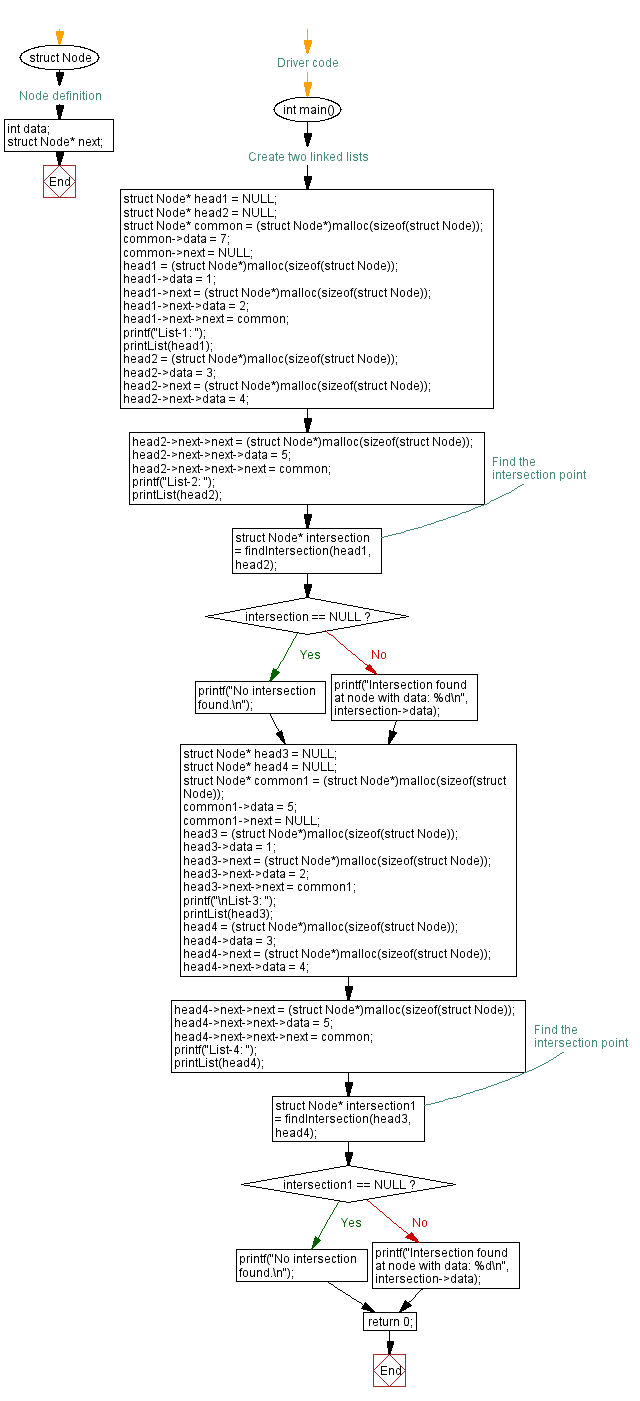﻿ C Program: Find the point at which two singly linked lists intersect

# C Exercises: Find the point at which two singly linked lists intersect

## C Singly Linked List : Exercise-42 with Solution

Write a C program to find the point at which two singly linked lists intersect.

Sample Solution:

C Code:

``````#include<stdio.h>
#include <stdlib.h>

// Node definition
struct Node {
int data;
struct Node* next;
};

// Function to find the length of a linked list
int len = 0;
len++;
}
return len;
}

// Function to find the intersection point of two linked lists
// Find the lengths of the linked lists

// Traverse the longer linked list by the difference in length
if (len1 > len2) {
int diff = len1 - len2;
for (int i = 0; i < diff; i++) {
}
} else if (len2 > len1) {
int diff = len2 - len1;
for (int i = 0; i < diff; i++) {
}
}

// Traverse both lists until we find a node that is present in both lists
}
}

// If no intersection is found, return NULL
return NULL;
}

}
printf("\n");
}

// Driver code
int main() {
struct Node* common = (struct Node*)malloc(sizeof(struct Node));
common->data = 7;
common->next = NULL;
printf("List-1: ");
printf("List-2: ");
// Find the intersection point
if (intersection == NULL) {
printf("No intersection found.\n");
} else {
printf("Intersection found at node with data: %d\n", intersection->data);
}

struct Node* common1 = (struct Node*)malloc(sizeof(struct Node));
common1->data = 5;
common1->next = NULL;
printf("\nList-3: ");
printf("List-4: ");
// Find the intersection point
if (intersection1 == NULL) {
printf("No intersection found.\n");
} else {
printf("Intersection found at node with data: %d\n", intersection->data);
}
return 0;
}
```
```

Sample Output:

```List-1: 1 2 7
List-2: 3 4 5 7
Intersection found at node with data: 7

List-3: 1 2 5
List-4: 3 4 5 7
No intersection found.
```

Flowchart :C Programming Code Editor:

What is the difficulty level of this exercise?

﻿